# Excel modeling Assignment Help

Using standard formula for pressure and substitute xB = 1 – xT into the pressure equation,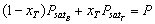and solve for xT,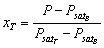(1)

## Excel modeling Assignment Help Through Online Tutoring and Guided Sessions from AssignmentHelp.Net

where the partial pressures are computed as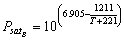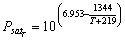The solution then consists of maximizing Eq. 1 by varying T subject to the constraint that 0 £ xT £ 1. The Excel Solver can be used to obtain the solution. Here is how the worksheet can be set up: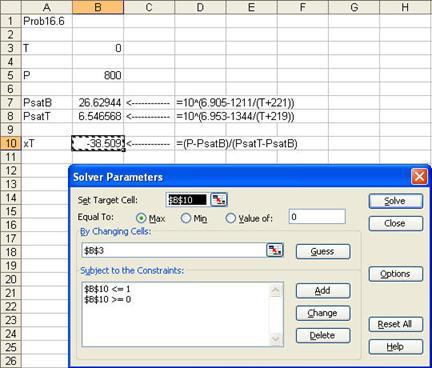The result is T = 112.8592 as shown below: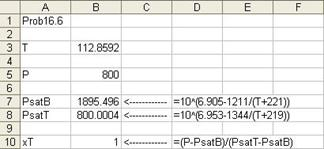Assignment Help | Excel Assignment Help | Excel Homework Help | Online Tutoring